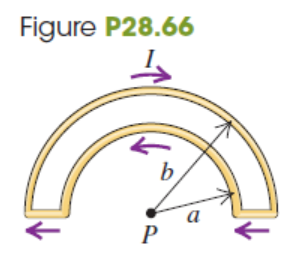# Problem: The wire semicircles shown in Fig. P28.66  have radii a and b. Calculate the net magnetic field (magnitude and direction) that the current in the wires produces at the point P.

###### FREE Expert Solution

Magnetic field:

$\overline{){\mathbf{B}}{\mathbf{=}}\frac{{\mathbf{\mu }}_{\mathbf{0}}\mathbf{i}}{\mathbf{4}\mathbf{r}}}$

The magnetic field due to the inner semicircle at point P is coming out of the page and is found as:

B2 = μ0i/4a

95% (1 ratings)###### Problem Details

The wire semicircles shown in Fig. P28.66  have radii a and b. Calculate the net magnetic field (magnitude and direction) that the current in the wires produces at the point P.